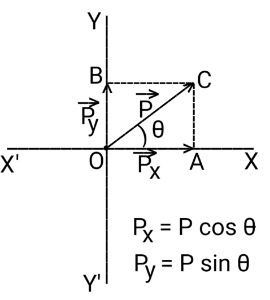# What are: (a) the x component and (b) the y component of a vector \vec{a} in the xy plane if its...

## Question:

What are:

(a) the x component and

(b) the y component of a vector {eq}\vec{a} {/eq} in the xy plane if its direction is {eq}238^{\circ} {/eq} counterclockwise from the positive direction of the x-axis and its magnitude is 9.6 m?

## Resolutes of a Vector:

A vector quantity is a physical quantity that can be characterized by a magnitude and a direction.

Displacement, velocity, acceleration, force, momentum, torque, etc are examples of vector quantities that will have both a magnitude and a direction each.

A vector is often graphically represented for better understanding.

A vector in the XY plane can be resolved into two vectors that are two mutually perpendicular components - one along the X-axis and the other along the Y-axis.Let {eq}\vec P {/eq} be represented by {eq}OC {/eq} directed from {eq}O \ \text{to} \ C {/eq} with {eq}O {/eq} being the origin.

The angle that the {eq}\vec P {/eq} makes with the +ve x-direction is {eq}\theta {/eq}.

The magnitude of the component of {eq}\vec P {/eq} along X-axis will be given by:

$$\displaystyle{P_x = P \ \cos \theta}$$.

The magnitude of the component of {eq}\vec P {/eq} along Y-axis will be given by:

$$\displaystyle{P_y = P \ \sin \theta}$$.

Given

• The magnitude of {eq}\vec a {/eq}: {eq}a = 9.6 \ \rm m {/eq}.
• The angle that {eq}\vec a {/eq} makes above +ve redirection counterclockwise: {eq}\theta = 238^\circ {/eq}.

Therefore, the magnitude of the x component of {eq}\vec a {/eq} will be given by:

\displaystyle{\begin{align*} a_x &= a \ \cos \theta\\ a_x &= (9.6 \ \rm )\times \cos (238^\circ)\\ a_x &\approx \boxed{{-5.1 \ \rm m}}\\ \end{align*}}

The magnitude of the y-component of {eq}\vec a {/eq} will be given by:

\displaystyle{\begin{align*} a_y &= a \ \sin \theta\\ a_y &= (9.6 \ \rm )\times \sin (238^\circ)\\ a_y &\approx \boxed{{-8.1 \ \rm m}}\\ \end{align*}}